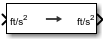# Acceleration Conversion

Convert from acceleration units to desired acceleration units

•Libraries:
Aerospace Blockset / Utilities / Unit Conversions

## Description

The Acceleration Conversion block computes the conversion factor from specified input acceleration units to specified output acceleration units and applies the conversion factor to the input signal.

The Acceleration Conversion block port labels change based on the input and output units selected from the Initial unit and Final unit parameters.

## Ports

### Input

expand all

Acceleration, specified as a scalar or array, in initial acceleration units.

#### Dependencies

The input port label depends on the Initial unit setting.

Data Types: `double`

### Output

expand all

Acceleration, returned as a scalar or array, in final acceleration units.

#### Dependencies

The output port label depends on the Final unit setting.

Data Types: `double`

## Parameters

expand all

Input units, specified as:

 `m/s`2 Meters per second squared `ft/s`2 Feet per second squared `km/s`2 Kilometers per second squared `in/s`2 Inches per second squared `km/h-s` Kilometers per hour per second `mph-s` Miles per hour per second `G's` g-units

#### Dependencies

The input port label depends on the Initial unit setting.

#### Programmatic Use

 Block Parameter: `IU` Type: character vector Values: `'ft/s^s` | `'m/s^2'` | `'km/s^2'` | `'in/s^2'` | `'km/h-s'` | `'mph/s'` | `'G's'` Default: `'ft/s2'`

Output units, specified as:

 `m/s`2 Meters per second squared `ft/s`2 Feet per second squared `km/s`2 Kilometers per second squared `in/s`2 Inches per second squared `km/h-s` Kilometers per hour per second `mph-s` Miles per hour per second `G's` g-units

#### Dependencies

The output port label depends on the Final unit setting.

#### Programmatic Use

 Block Parameter: `OU` Type: character vector Values: `'ft/s^2'` | `'m/s^2'` | `'km/s^2'` | `'in/s^2'` | `'km/h-s'` | `'mph/s'` | `'G's'` Default: `'ft/s2'`

## Version History

Introduced before R2006a